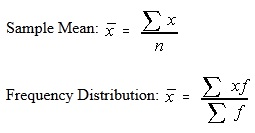#### Concept of Measures of Central Tendency

Measures of Central Tendency:

The term ‘Average’ is not clear:

The term Average could mean one of four things: Arithmetic mean, Median, Midrange and the mode. For the above reason, the type of average must be stated prior.

Mean:

People usually intend the word average by the term Mean.

Population Mean: μ = (Σ x)/NThe weighted mean can also be termed by the mean of a frequency distribution

Median:

At first the data must be sorted in ascending order (or ranked). Median is also defined as the number in the middle.

There are some formulas which could be used to determine the depth of median. The one of them is as follows:

Depth of median = 0.5 * (n + 1)

Raw Data:

The median is a number in the ‘depth of the median’ place. If the size of the sample is even then the depth of median will be a decimal. You require finding the midpoint between the numbers on either side of the depth of median.

Ungrouped Frequency Distribution:

At first determine the cumulative frequencies for the data. The very first value with cumulative frequency more than depth of median is the median. And if the depth of median is precisely 0.5 greater than the cumulative frequency of the prior class, then median is the midpoint among two classes.

Grouped Frequency Distribution:

This distribution is quite hard.

In this we have lost all original information because the data is grouped. Some of the textbooks have simply taken the midpoint of class. This is an over-simplification that isn't the true value (save for much simpler to do). Interpolate is the precise procedure.
Then find out in what proportion the distance to the median class is, after that divide the median of sample size by 2, subtracting the cumulative frequency of the prior class, and then dividing that entire bay the frequency of median class.

Multiply this proportion by the class width and sum it to the lower boundary of median class.

MD = x = [(n/2 - cf)/f]w + Lm

Mode:

The mode is most recurrent data value. There might be no mode when no one value appears greater than any other. There might also be two modes (that is bimodal): three modes (trimodal) or more than three modes (multi-modal).

The modal class is a class with the maximum frequency in grouped frequency distributions.

Midrange:

The midpoint of highest and lowest values is simply stated as the midrange.

Summary:

The Mean is utilized in computing other statistics (like the variance) and doesn’t exist for open ended grouped frequency distributions. It is frequently not suitable for skewed distributions like salary information.

Median is the center number and is excellent for the skewed distributions as it is resistant to change.

The Mode is employed to explain the most typical case. The mode can be utilized with nominal data while the others cannot. The mode might or might not exist and there might be more than one value for the mode.

The Midrange is not very frequently used. This is a very rough estimate of average and is very much affected by the extreme values.

 Property Mean Median Mode Midrange Always Exists No Yes No Yes Uses all data values Yes No No No Affected by extreme values Yes No No Yes

Latest technology based Statistics Online Tutoring Assistance

Tutors, at the www.tutorsglobe.com, take pledge to provide full satisfaction and assurance in Statistics help via online tutoring. Students are getting 100% satisfaction by online tutors across the globe. Here you can get homework help for Statistics, project ideas and tutorials. We provide email based Statistics help. You can join us to ask queries 24x7 with live, experienced and qualified online tutors specialized in Statistics. Through Online Tutoring, you would be able to complete your homework or assignments at your home. Tutors at the TutorsGlobe are committed to provide the best quality online tutoring assistance for Statistics Homework help and assignment help services. They use their experience, as they have solved thousands of the Statistics assignments, which may help you to solve your complex issues of Statistics. TutorsGlobe assure for the best quality compliance to your homework. Compromise with quality is not in our dictionary. If we feel that we are not able to provide the homework help as per the deadline or given instruction by the student, we refund the money of the student without any delay.